Question

A volcanic plume puts ash into the atmosphere to altitude of 10000m. The characteristic ash particle...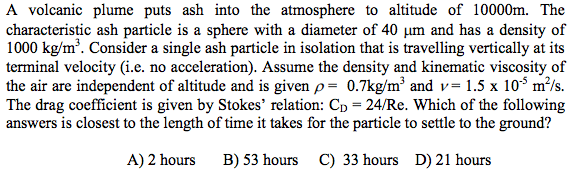A volcanic plume puts ash into the atmosphere to altitude of 10000m. The characteristic ash particle is a sphere with a diameter of 40 mum and has a density of 1000 kg/m^3. Consider a single ash particle in isolation that is travelling vertically at its terminal velocity (i.e. no acceleration). Assume the density and kinematic viscosity of the air are independent of altitude and is given rho = 0.7 kg/m^3 and nu = 1.5 times 10^-5 m^2/s. The drag coefficient is given by Stoke's relation: C_D = 24/Re. Which of the following answers is closest to the length of time it takes for the particle to settle to the ground? 2 hours 53 hours 33 hours 21 hours

Solution:

Settling velocity is used to determine the approximate time for the ash particle to settle down from 10000m,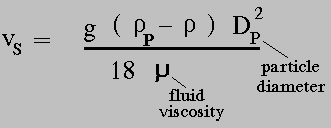Vs = (9.81* (1000-0.7)* 40 E-06 ) / 18 = 0.02178 m/s

so it takes 33 hours approx for the ash particle to settle down

Option (B) 53 hours.

Earn Coins

Coins can be redeemed for fabulous gifts.

Similar Homework Help Questions
• A volcanic plume puts ash into the atmosphere to altitude of 10000m. The characteristic ash particle...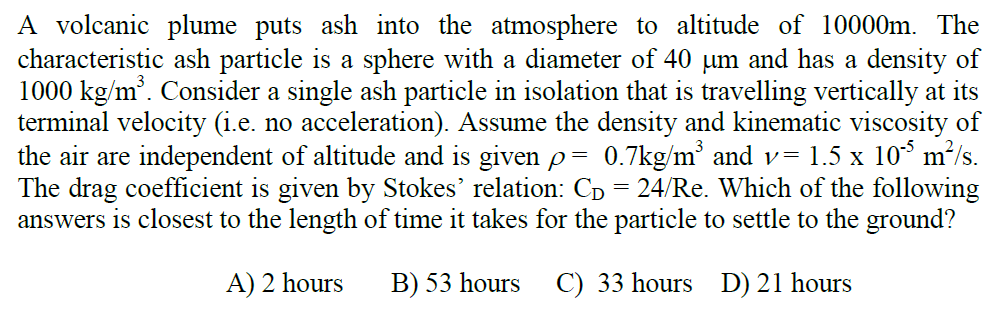A volcanic plume puts ash into the atmosphere to altitude of 10000m. The characteristic ash particle is a sphere with a diameter of 40 um and has a density of 1000 kg/m3. Consider a single ash particle in isolation that is travelling vertically at its terminal velocity (i.e. no acceleration). Assume the density and kinematic viscosity of the air are independent of altitude and is given ,-07kg/m3 and v= 1.5 x 10° m2/s. The drag coefficient is given by Stokes'...

• Determine the residence time (in hours) for a 25-um particle in the atmosphere with a density of 1,200 kg/m3 at an elevation of 3,500 m. Assume the gravitational acceleration is 9.8 m/s2 and the...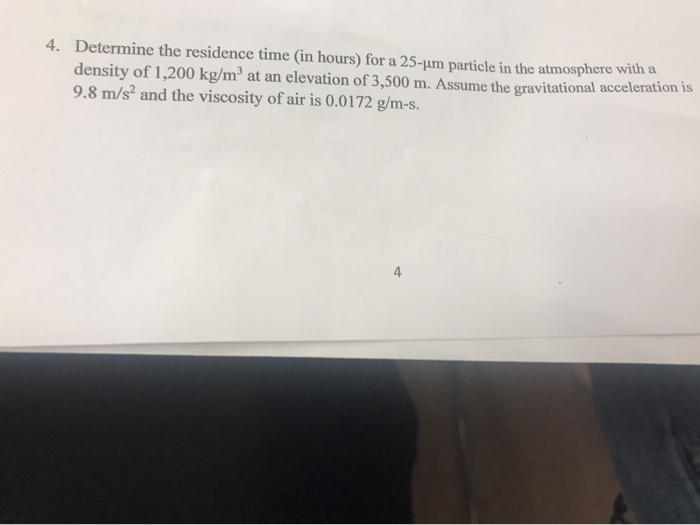Determine the residence time (in hours) for a 25-um particle in the atmosphere with a density of 1,200 kg/m3 at an elevation of 3,500 m. Assume the gravitational acceleration is 9.8 m/s2 and the viscosity of air is 0.0172 g/m-s. 4. 4 Determine the residence time (in hours) for a 25-um particle in the atmosphere with a density of 1,200 kg/m3 at an elevation of 3,500 m. Assume the gravitational acceleration is 9.8 m/s2 and the viscosity of air is...

• Lecture I1.3. Drag Force 267 26 percent of greenhouse gas emissions come from cars, with a typica...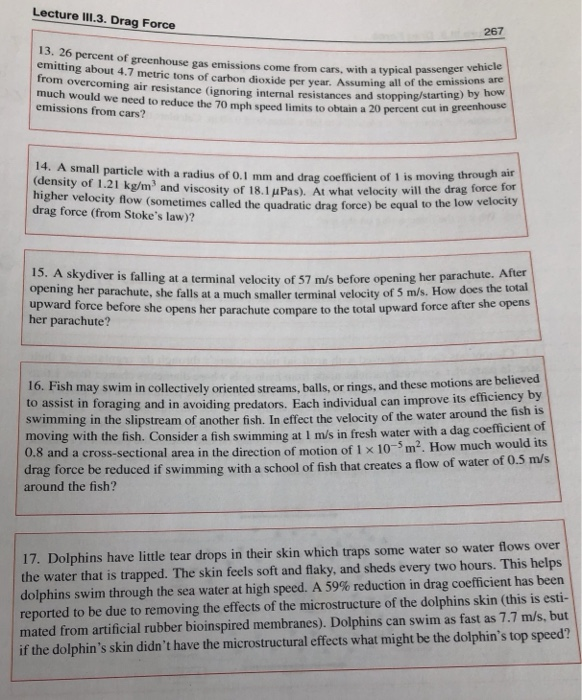Lecture I1.3. Drag Force 267 26 percent of greenhouse gas emissions come from cars, with a typical passeng emitting about 4.7 metric tons of carbon dioxide per year. Assuming all of the rom overcoming air resistance (ignoring internal resistances and stopping/starting much would we need to reduce the 70 mph speed limits vehicle emissions are how to obtain a 20 percent cut in greenhouse er emissions from cars? 14. A small particle with a radius of 0.1 mm and drag...